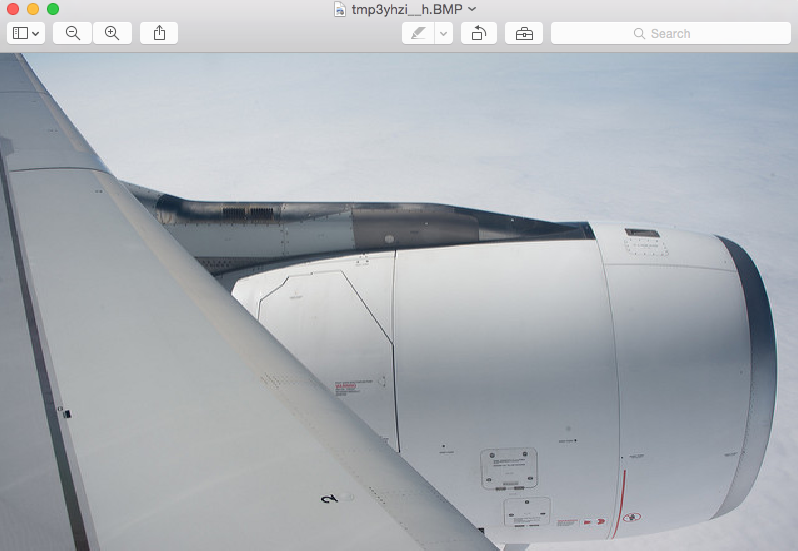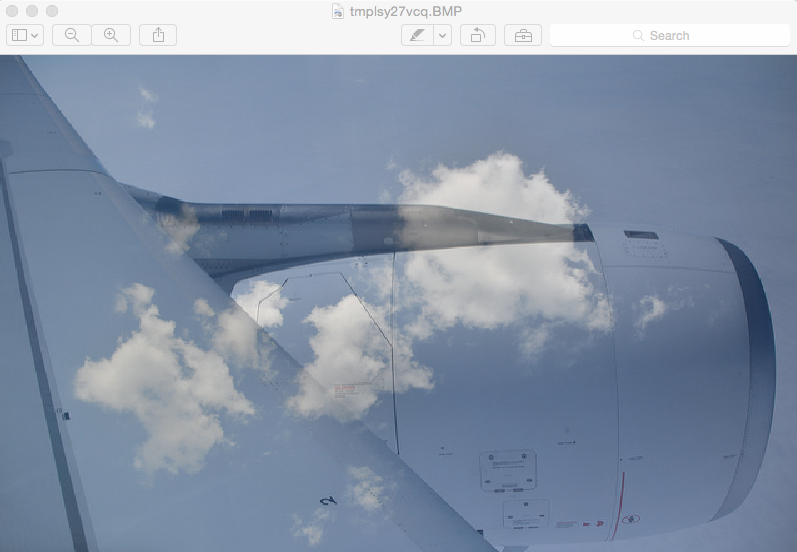# Alpha Composite Of Two Images In Pillow

## Overview:

• An alpha channel of an image represents its transparency.

• Pillow the Python Image Processing Library provides alpha compositing of images through the Image class method alpha_composite().

• When the value of alpha channel of one image is 0 - and of another image is 1, the alpha_composite() will display only the second image since its pixels are opaque.

• When the value of alpha channel of one image is 1 - and of another image is 0, the alpha_composite() will display only the first image as the pixels of the first image are opaque.

• When alpha channels of both the images are opaque, both the pixels are to be displayed - Transparency levels are determined by the color of the pixel's' from both the images equally.

## Example:

 from PIL import Image # Function to change the image size def changeImageSize(maxWidth,                      maxHeight,                      image):          widthRatio  = maxWidth/image.size     heightRatio = maxHeight/image.size     newWidth    = int(widthRatio*image.size)     newHeight   = int(heightRatio*image.size)     newImage    = image.resize((newWidth, newHeight))     return newImage # Take two images     image1 = Image.open("./sky.png") image2 = Image.open("./plane.png") # Make the sizes of images uniform image3 = changeImageSize(800, 500, image1) image4 = changeImageSize(800, 500, image2) # Make sure the images have alpha channels image3.putalpha(1) image4.putalpha(1) # Display the images image3.show() image4.show() # Do an alpha composite of image4 over image3 alphaComposited = Image.alpha_composite(image3, image4) #alphaBlended = Image.blend(image4, image3,.1) #alphaBlended.show() # Display the alpha composited image alphaComposited.show()

## Image2 of the alpha composite:## The Alpha Composite of two images: